﻿ 用众数法和线性插值法及主成分分析法与字符串匹配法——对互联网时代的相亲的研究 Use of the Crowd-Count Method and Linear Interpolation Method and Principal Component Analysis Method and String Matching Method—Research on Blind Dates in the Internet Age

Computer Science and Application
Vol. 09  No. 03 ( 2019 ), Article ID: 29323 , 16 pages
10.12677/CSA.2019.93067

Use of the Crowd-Count Method and Linear Interpolation Method and Principal Component Analysis Method and String Matching Method

—Research on Blind Dates in the Internet Age

Maoyi Zhang, Haolin Jia, Lele Sun

School of Information and Control Engineering, China University of Mining and Technology, Xuzhou Jiangsu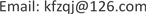Received: Feb. 25th, 2019; accepted: Mar. 13th, 2019; published: Mar. 20th, 2019ABSTRACT

The emergence of the Internet provides a new and quick way to find a blind date. In this paper, the data provided by matchmaking companies are modified and filled with the method of number and linear interpolation, and the mathematic model of the overall satisfaction of all matchmaking companies is established. The quality of the members of all matchmaking companies is sorted, and the data of male and female members are processed separately by using the principal component analysis method. Finally, the high-quality male members and high-quality female members are listed, which has a certain reference value for domestic matchmaking companies.

Keywords:Mass Method, Linear Interpolation, Principal Component Analysis, String Matching Method, Minimum Absolute Residual, Blind Date, Cloud Model Test

——对互联网时代的相亲的研究1. 概述

2. 研究的问题细化

2.1. 引言

21世纪人类进入了互联网时代，人们的物质条件相比过去都有了长足的发展，但是进入现代社会的剩男剩女却越来越多。现在电视上各种相亲节目层出不穷，非常火爆，各种商业性的婚介机构也相继出现。目前市场上的婚介机构运作模式大都是单身男女交纳一定数量的费用成为会员，然后由机构的专业人员将注册会员的信息进行逐项比对，将匹配度较高的单身男女进行配对后，通知双方相亲。但由于注册会员的人数往往数量庞大，仅靠人工进行配对，不仅会存在很多的人为局限性，错过许多良缘，而且工作效率低下。现需要研究某婚恋网站的信息配对系统，如何使该婚恋软件的到更好得被使用 。

2.2. 问题的提出

3. 问题分析

3.1. 问题一的分析

3.2. 问题二的分析

3.3. 问题三的分析

4. 模型假设

1) 男女会员的所有数据均真实无误。

2) 男女会员的缺失数据为随机缺失。

3) 男女会员在填写配偶要求时，会根据自身的条件去考虑，如女性会员会挑选比自己高的会员。

4) 假设单位类型一项并无前后质量的区别。

5) 假设会员所拥有的房子价值相同。

6) 假设大部分男女会员的婚恋观符合常人的婚恋观念，拥有正确主流的价值观念。

5. 符号说明

6. 模型的建立与求解

6.1. 问题一模型的建立与求解

6.1.1. 数据的修正

1) 在会员身高一栏处，有1.77 cm的数据，这是误填，将其改为177 cm；有部分数据为0，将其改为空缺值。

2) 在会员和其要求对象的文化程度的数据中有“请选择”，不符合常理，将其改为空缺值。还有数据显示为“不限”，我们通过筛选发现这些数据很少，并不会影响整体大样本的分析，故我们决定也将其删去作为空缺值。

3) 在会员收入中出现了诸多“2~3000”，“2000实习”等字样，这些对处理数据都无法实现，我们将其取为平均值，实现数据的相对离散。在会员要求对象的数，这些均不符合常理，同意改为空缺值。

4) 在年龄差上限和下限中，存在正数负数相间分布的现象，并且对于超过20的数据经分析应该是会员要求对方的实际年龄，为避免混乱，我们将年龄差定义为“会员要求对象的实际年龄–会员自己的实际年龄”，将数据进行清洗。

6.1.2. 数据的填补Table 3. Quantitative table of member requirements for spouses

1) 对于“婚姻状况”，“文化程度”，“住房情况”，“地区”，“单位类型”数据，这些数据的共同特征在于，对它们进行量化编码之后，其数字都在0到9之间。经分析之后，会发现其中某一个数字出现的频率在60%到70%之间，为此我们采用众数的填补方法。因为出现频率最高的数字，比例已经过半，所以对于部分空白的填补并不会影响整个数据的分析。通过众数填补方法，可以比较客观的反映这些指标的分布状况。

2) 对于“会员收入”和“会员要求对象的收入”的数据，这两组数据的特征在于它们与其他数据之间的关系，并不存在明显的函数关系。他们的数字，范围比较广，因此不能采用众数的填补方法。因此可以采取线性插值的方法来填补这些数据的空缺值，插值的方法，是将所有数据的点尽可能控制在一条曲线之内，这样可以避免奇异值出现。因此该数据能比较真实的反映输入的真实情况。而后我们将填补后的数据，进行整合分析，如图1图2所示，通过图形，我们可以发现，无论是“会员收入”还是“会员要求对象的收入”这两栏，没有出现很大的出入，“会员要求对象的收入”总是与“会员收入”基本相一致。在不同的区间都存在一定数量的样本，存在部分过高或过低的值，但是仍然有一定的比例。因此，这种方法实现填补空缺是合理的。会员自身收入分布图见图1；要求配偶收入分布图见图2 。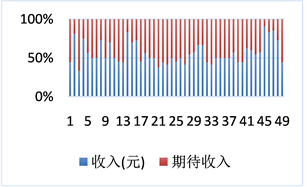Figure 1. Distribution of members’ own income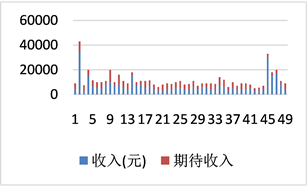Figure 2. Distribution of spousal income required

3) 考虑到一般情况下，会员自身身高与对配偶身高的期望值，即身高上下限，有一定的影响，例如自身身高偏高，就很可能选择身高较高的配偶，或者有些男性会员会要求配偶身高不能高于自己。对于男性女性的择偶标准中的身高上下限的数据缺失部分，可以用数据完整的会员自身身高与其要求的身高上下限所拟合得到的回归曲线进行预测。对已知数据的拟合可用最小绝对残差法对所选自变量——身高，与因变量——配偶身高上限或者下限，进行拟合得到拟合直线，该方法可剔除个别与全局偏差较多的数据，使残差最小，相比于简单的额最小二乘法更适用于该题目，用得到的拟合线性方程在excel中对身高上下限缺失的会员进行身高上下限的预测与计算，得到与之对应的身高上下限。男择女身高上限见图3；男择女身高下限见图4Figure 3. Male height ceiling chart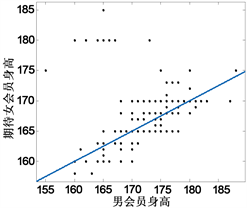Figure 4. Male height ceiling

$\left\{\begin{array}{l}{y}_{1}=0.5x+80\\ {y}_{2}=0.40x+90.19\\ {y}_{3}=0.028x+175.4\\ {y}_{4}=0.4x+106\end{array}$ (1)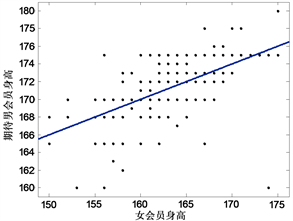Figure 5. Female height ceiling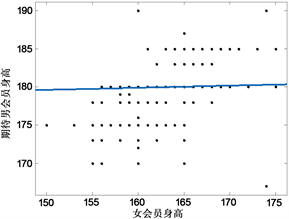Figure 6. Lower height of female elect maleFigure 7. Scatter of height, upper and lower limits for men and women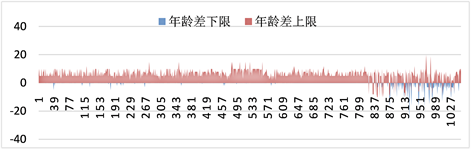Figure 8. Distribution of age difference

6.2. 问题二的模型建立与求解Figure 9. Step diagram of string matching method

6.2.1. 模型原理

$ratio=2×M/T$ (2)

6.2.2. 模型建立

1) 首先，数据中有些会员是无法找到其匹配程度的，这些会员没有相似度得分，经验证，366个会员无法进行匹配。

2) 接着我们要把可以匹配会员的候选人数找出来，以下表举例。部分会员符合标准异性数量表见表4。同时我们令集合为显示行矩阵，既将会员所匹配对象所在那一行显示出来。由于计算时所用行数与实际行数有一定差别，因此可能不同。部分会员符合标准异性数量表见表4Table 4. Some members meet the standard heterosexual scale

3) 进行反向判断Table 5. Partial member matching similarity results

6.2.3. 模型分析

${S}_{i}=\left\{{\alpha }_{1},{\alpha }_{2},{\alpha }_{3},\cdots ,{\alpha }_{n}\right\}$ (3)

${\gamma }_{i}$ 为第i个会员的相似度得分最大值

${r}_{i}=\mathrm{max}\left\{{\alpha }_{1},{\alpha }_{2},{\alpha }_{3},\cdots ,{\alpha }_{n}\right\}$ (4)

$\beta ={r}_{1}+{r}_{2}+\cdots +{r}_{i}$ (5)

6.3. 问题三的模型建立与假设

6.3.1. 模型分析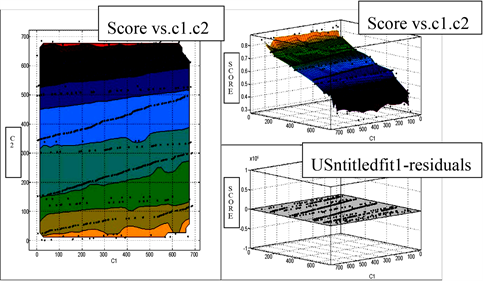Figure 10. Distribution of member matching resultsFigure 11. The steps of component analysis

PCA的原理是通过分析得到两个或更多的变量f1，f2能够尽可能反映原来多个变量的信息，也就是说它的方差尽量大，而且f2中的信息并不和f1中所代表的影响因素所重合，即Cov(f1, f2) = 0，Cov表示协方差。主成分分析法已经是一种比较成熟的方法，在此，我们给出简要的分析步骤如图11

6.3.2. 模型建立过程

1) 权重的确定Table 6. Quantified by age segment for males and females

2) 数据标准化处理

Z-Score通过 $\left(x-\mu \right)/\sigma$ 将两组或多组数据转化为无单位的Z-Score分值，使得数据标准统一化，提高了数据可比性，削弱了数据解释性。这样处理后对于一些信息比如“住房情况”、“婚姻状况”、“文化程度”情况比较方便对比，从而得出相关系数矩阵。

3) 模型求解

Step 1得出相关系数矩阵

$R=\left[\begin{array}{cccc}{r}_{11}& {z}_{12}& ...& {z}_{1p}\\ {r}_{21}& {z}_{22}& ...& {z}_{2p}\\ ⋮& ⋮& ⋮& ⋮\\ {r}_{p1}& {z}_{p2}& ...& {z}_{pp}\end{array}\right]$ (6)

${r}_{ij}=\frac{\sum _{k=1}^{n}\left({x}_{ki}-{\overline{x}}_{i}\right)\left({x}_{kj}-{\overline{x}}_{j}\right)}{\sqrt{\sum _{k=1}^{n}{\left({x}_{ki}-{\overline{x}}_{i}\right)}^{2}\sum _{k=1}^{n}{\left({x}_{kj}-{\overline{x}}_{j}\right)}^{2}}}$ (7)

Step 2 计算各个主成分的贡献率Table 7. Male membership feature values and principal component contribution table

Step 3 计算主成分负荷

$Y=\left[\begin{array}{lllll}{Y}_{1}\hfill & {Y}_{2}\hfill & {Y}_{3}\hfill & {Y}_{4}\hfill & {Y}_{5}\hfill \end{array}\right]$ (8)

${Y}_{1}={X}_{1}{E}_{1}$ (9)

${Y}_{2}={X}_{2}{E}_{2}$ (10)

${E}_{1}=\left[\begin{array}{lllll}0.57\hfill & 0.11\hfill & 0.29\hfill & 0.17\hfill & 0.09\hfill \\ -0.28\hfill & 0.52\hfill & 0.13\hfill & 0.37\hfill & -0.62\hfill \\ 0.53\hfill & 0.20\hfill & 0.38\hfill & 0.15\hfill & -0.15\hfill \\ 0.37\hfill & 0.42\hfill & -0.28\hfill & -0.28\hfill & 0.26\hfill \\ -0.29\hfill & -0.10\hfill & 0.75\hfill & 0.06\hfill & 0.42\hfill \\ -0.24\hfill & 0.55\hfill & -0.19\hfill & 0.38\hfill & 0.57\hfill \\ -0.19\hfill & 0.43\hfill & 0.27\hfill & -0.76\hfill & -0.09\hfill \end{array}\right]{E}_{2}=\left[\begin{array}{lllll}0.70\hfill & -0.04\hfill & 0.09\hfill & 0.21\hfill & 0.01\hfill \\ 0.07\hfill & 0.21\hfill & 0.44\hfill & -0.79\hfill & -0.33\hfill \\ 0.70\hfill & -0.14\hfill & 0.01\hfill & -0.11\hfill & -0.08\hfill \\ 0.05\hfill & 0.61\hfill & 0.09\hfill & -0.11\hfill & 0.68\hfill \\ -0.14\hfill & -0.33\hfill & 0.57\hfill & 0.06\hfill & 0.53\hfill \\ 0.05\hfill & 0.67\hfill & 0.04\hfill & 0.35\hfill & -0.22\hfill \\ -0.04\hfill & 0.02\hfill & 0.68\hfill & 0.42\hfill & -0.31\hfill \end{array}\right]$

${X}_{1}=\left[\begin{array}{lllllll}{x}_{11}\hfill & {x}_{12}\hfill & {x}_{13}\hfill & {x}_{14}\hfill & {x}_{15}\hfill & {x}_{16}\hfill & {x}_{17}\hfill \end{array}\right]$ (11)

${X}_{2}=\left[\begin{array}{lllllll}{x}_{21}\hfill & {x}_{22}\hfill & {x}_{23}\hfill & {x}_{24}\hfill & {x}_{25}\hfill & {x}_{26}\hfill & {x}_{27}\hfill \end{array}\right]$ (12)

Step 4 得出综合评价系数

${P}_{1}=0.28726×{Y}_{11}+0.20462×{Y}_{12}+0.15171×{Y}_{13}+0.12612×{Y}_{14}+0.0934×{Y}_{15}$ (13)

${P}_{2}=0.19961×{Y}_{21}+0.16754×{Y}_{22}+0.16299×{Y}_{23}+0.13875×{Y}_{24}+0.13043×{Y}_{25}$ (14)

6.3.3. 结果分析与验证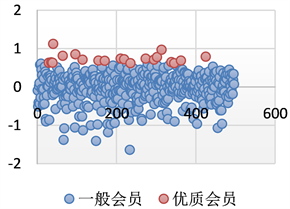Figure 12. Distribution of quality male members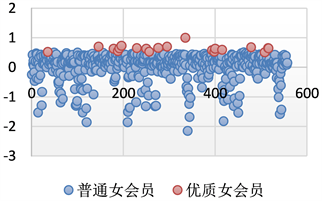Figure 13. Distribution of quality female members

6.3.4. 模型灵敏度分析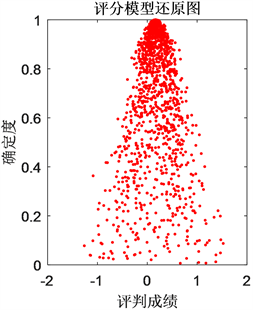Figure 14. Females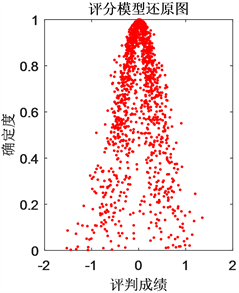Figure 15. Male

7. 模型的评价与推广

7.1. 模型的评价

7.1.1. 模型的优点

1) 在求解第一问时，我们运用两种方法分别对身高差值上下限和期待对象收入确实数据进行填补，即最小绝对残差法和线性插值法。因为在挑选对象身高时，会员会结合自身身高作为参考，所以运用两种方法填补数据比较符合实际，得出结果更加细致准确。

2) 在求解第二问时，我们运用的是字符串长度匹配的方法，这是一种古老的、研究广泛的计算机匹配算法。运用Python编程，当一个会员的自身条件与另一个会员期待的条件相似度最大时，即可认为满意度最大，这种方法运用起来简单。

3) 在求解第三问时，我们结合实际情况，优质男女会员的评价指标不尽相同，比如年龄在评价优质女会员时占较大比重。于是我们运用主成分分析方法将男女会员数据分别进行分析得到各自的主成分，这样评价较为客观。

7.1.2. 模型的缺点

8. 结束语

Use of the Crowd-Count Method and Linear Interpolation Method and Principal Component Analysis Method and String Matching Method—Research on Blind Dates in the Internet Age[J]. 计算机科学与应用, 2019, 09(03): 588-603. https://doi.org/10.12677/CSA.2019.93067

1. 1. 宋海龄, 蔡季冰. 最小绝对残差和实用算法的研究与应用[J]. 信息与控制, 1988(3): 60-62.

2. 2. 胡庆婉. 使用MATLAB曲线拟合工具箱做曲线拟合[J]. 电脑知识与技术, 2010, 6(21): 5822-5823.

3. 3. 李雪莹, 刘宝旭, 许榕生. 字符串匹配技术研究[J]. 计算机工程, 2004, 30(22): 24-26.

4. 4. 李连香, 许迪, 程先军, 李晓琴, 余和俊. 基于分层构权主成分分析的皖北地下水水质评价研究[J]. 资源科学, 2015, 37(1): 61-67.

5. 5. 林海明, 张文霖. 主成分分析与因子分析的异同和SPSS软件——兼与刘玉玫、卢纹岱等同志商榷[J]. 统计研究, 2005(3): 65-69.